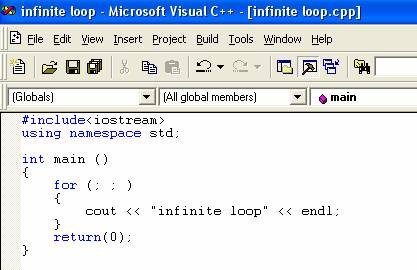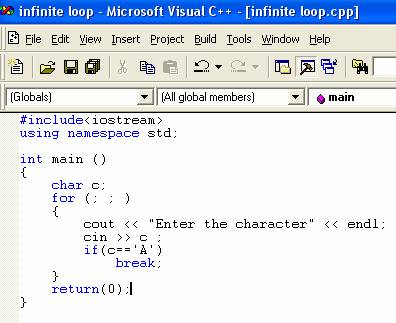Loops

Before reading this tutorial, you should have knowledge of switch statements.

Loops are iteration statements. Loop provides a mechanism to execute same sequence of instructions repeatedly until a condition is reached. There are two elements of the loop: the body of loop which is to be executed number of times and a loop condition which terminates the loop when a particular condition is met.  The three different kinds of a loop are: -

• The for loop
• The while loop
• The do while loop

The for loop

The for loop is used to execute same sequence of statements for the predetermined number of times. The general form of the for loop is: -

for(initialization; condition; iteration_expression)

{

statement;

}

The initialization is used to initialize the control variable of the loop. The initialization is executed once at the beginning of the loop. The condition is checked at the beginning of every iteration and if the condition is false then the loop is terminated. The iteration_expression is executed at the end of each loop iteration. It modifies the control variables of the loop initialized in the beginning.  Here is a program which illustrates the working of the for loop.The result of the program is: -The program is used to calculate the sum of first 10 numbers using a loop. There are 10 iterations of the loop. The variable c is initialized to zero. In the statement

for (int i=1; i<=10; i++)

i=1; is the initialization expression which sets the value of control variable to be 1. The condition is

i<=10;

and at the beginning of each iteration  this condition is checked whether the value of variable i exceeds value 10 . The iteration_expression is

i++;

and at the end of each iteration the value if variable i  is incremented by 1.  In the body of the loop the statement

cout << i << endl;

prints the value of  i for every iteration and the statement

c=c+i;

computes the sum of the values of the variable i. At the last iteration the value of the variable i becomes 11 when statement i++; is executed and then the condition i<=10; is checked and now the condition is false and as a result the loop is terminated. The control of the program is transferred to the statement following the for loop. The statement

cout << “The sum is” << c << endl;

is executed and which prints the sum as 55.

In the for loop, the body of the loop is never executed if the condition is false at the beginning of the first iteration. There are many variations of the for loop. User can use comma operator to declare two or more variables to control the loop. For example,

for(int i=0 , int j=0; i+j<=10; i++)

j++;

variables i and j are controlling the loop. The statement int i=0, int j=0; are initialization statements.

The Infinite loop

The for loop can be used to create infinite loop. The infinite loop can be made by leaving the conditional expression empty. Here is the program of  the infinite loop .The statement

cout << “infinite loop” << endl;

is executed infinite times as there is no condition expression in the for statement. The infinite loop can be broken using an if and break statement. Here is the program which shows how to discontinue the infinite loop.The result of the program is:-In each iteration the user is allowed to enter a character by using the statement

cin >> c;

The statement

If(c==’A’)

checks whether the character entered is A. If the character is ‘A’ the loop terminates with the help of statement

break;

If the character is not ‘A’ loop continues.  The loop will continue as long as user does not enter character ‘A’.

Go to the previous lesson or proceed to the next lesson